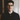Enea Xharja

# JavaScript Operators

Numeric data in JavaScript is represented by the `Number` data type.

In JavaScript, like in mathematics, the `+` symbol is used as an addition operator when in between two number.

Example:

``var randomNumber = 7 + 10; // 17``

## Subtract One Number from Another

JavaScript uses the `-` symbol for subtraction, in order to subtract one number from another:

``var randomNumber = 10 - 5; // 5``

## Multiply Two Numbers

JavaScript uses the `*` symbol for multiplication of two numbers:

``var randomNumber = 10 * 10; // 100``

## Divide One Number by Another

JavaScript uses the `/` symbol in order to divide one number by another:

``var randomNumber = 100 / 10; // 10``

## Increment a Number

In JavaScript, we can use the `++` operator to increment or add one to a variable:

``i++;``

is the equivalent of

``i = i + 1;``

## Decrement a Number

In JavaScript, we can use the `--` operator to decrement or decrease a variable value by one:

``i--;``

is the equivalent of

``i = i - 1;``

## Create Decimal Numbers

Variables can store also decimal number, which are referred to also as `floating point numbers` or simply `floats`.

``var myDecimal = 9.9;``

## Multiply Two Decimals

Just like with whole numbers, in JavaScript, we can perform calculations also with decimal numbers:

``var product = 2.0 * 2.5; // 5``

## Divide One Decimal by Another

Now let’s divide one decimal by another:

``var quotient = 6.6 / 2.0; // 3.3``

## Finding a Remainder

In programming, it’s common to check if a number is `even` (divisible by 2) or `odd` (not divisible by 2).

The remainder operator `%` gives the remainder of the division of two numbers:

``````17 % 2 = 1; // 17 ==> Odd
10 % 2 = 0; // 10 ==> Even``````

We can break this down by:

``````Math.floor(17 / 2) = 8;
8 * 2 = 16;
17 - 16 = 1; // Remainder``````

## Compound Assignment With Augmented Addition

In programming, we use assignments to modify the content of a variable. Moreover, other than assigning a new value, we saw also how to perform basic mathematical operation.

In JavaScript, we can combine a mathematical operator and the assignment operator and obtain a new operator used for augmented addition `+=`:

``````var myFavoriteNumber = 1;
myFavoriteNumber += 16;
console.log(myFavoriteNumber); // 17``````

## Compound Assignment With Augmented Subtraction

Just like the `+=` operator we saw before, `-=` subtracts a number from a variable:

``````var myFavoriteNumber = 4;
myFavoriteNumber -= 1;
console.log(myFavoriteNumber); // 3``````

## Compound Assignment With Augmented Multiplication

Using the `*=` operator, we can multiply a variable by a number:

``````var myFavoriteNumber = 5;
myFavoriteNumber *= 6;
console.log(myFavoriteNumber); // 30``````

## Compound Assignment With Augmented Division

Using the `/=` operator, we can divide a variable by another number:

``````var myFavoriteNumber = 10;
myFavoriteNumber /= 2;
console.log(myFavoriteNumber); // 5``````

Updated on May 10, 2020

Tagged with:Hey there! I'm Enea, a Web Developer at wohnvoll in Berlin. Welcome to my little corner of the web, where I share my personal collection of notes, code snippets, and resources on topics that interest me.